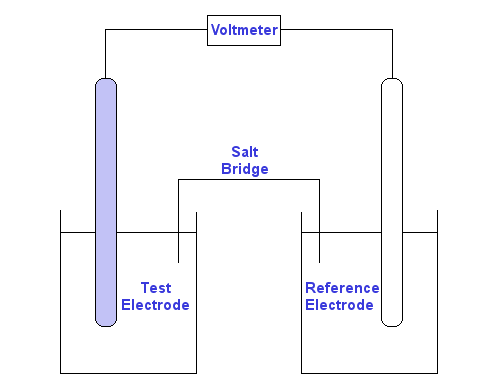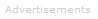# Definition of Standard Redox Potential

What is a Standard Redox Potential?

A standard redox potential, symbol Eo, is the electric potential of an electrochemical half-cell relative to a standard electrochemical half-cell under standard conditions.

Standard redox potential is also known as the standard reduction potential.

### Measuring Standard Redox Potentials

Standard redox potentials are measured relative to a standard hydrogen electrode, SHE, which is assigned a standard redox potential of 0 volts.Measuring a Standard Redox Potential, Eo, relative to a SHE reference electrode.

The conditions for measurement of a standard redox potential are:

• Temperature: 25 °C (298.15 K)
• Pressure: 1 atm (101.325 kPa)
• Solute Concentration: 1 mol dm-3 (1 M)

Use of a high impedance voltmeter ensures negligible electric current flows, therefore standard redox potentials are measured under reversible/equilibrium conditions.

### Non-Standard Conditions

Redox potentials measured under non-standard conditions are identified by the symbol E rather than Eo.

For example, if an experiment to find the effect of concentration on a half-cell's behavior gathered data at concentrations different from 1 M, measurements would be redox potentials E rather than standard redox potentials Eo.

### Interpreting the Standard Redox Potential's Sign ±

Standard redox potentials are always written as reductions, even if the reaction that actually took place was an oxidation. The half-cells with the greatest tendency to reduction are assigned positive potentials, while those with the greatest tendency to oxidation have negative potentials.

For example, the standard redox potential for fluorine, which has a very high affinity for electrons, is positive. The reduction potential for lithium, which is highly likely to lose electrons, is negative:

F2(g)  +  2e-  ⇌   2F-    Eo = +2.87 V
Li+   +  e-  ⇌  Li         Eo = -3.04 V

Eo data can be used to predict the voltages available from electric cells.

### Gibbs Free Energy

Eo can also be used to calculate the Gibbs free energy ΔGo for a half-reaction using the equation:

ΔGo = -nFEo

where n is the number of moles of electrons transferred in the half-reaction equation and F is the Faraday constant.

### Biological Measurements

In biological systems the standard redox potential is defined at pH = 7.0.Search the Dictionary for More Terms